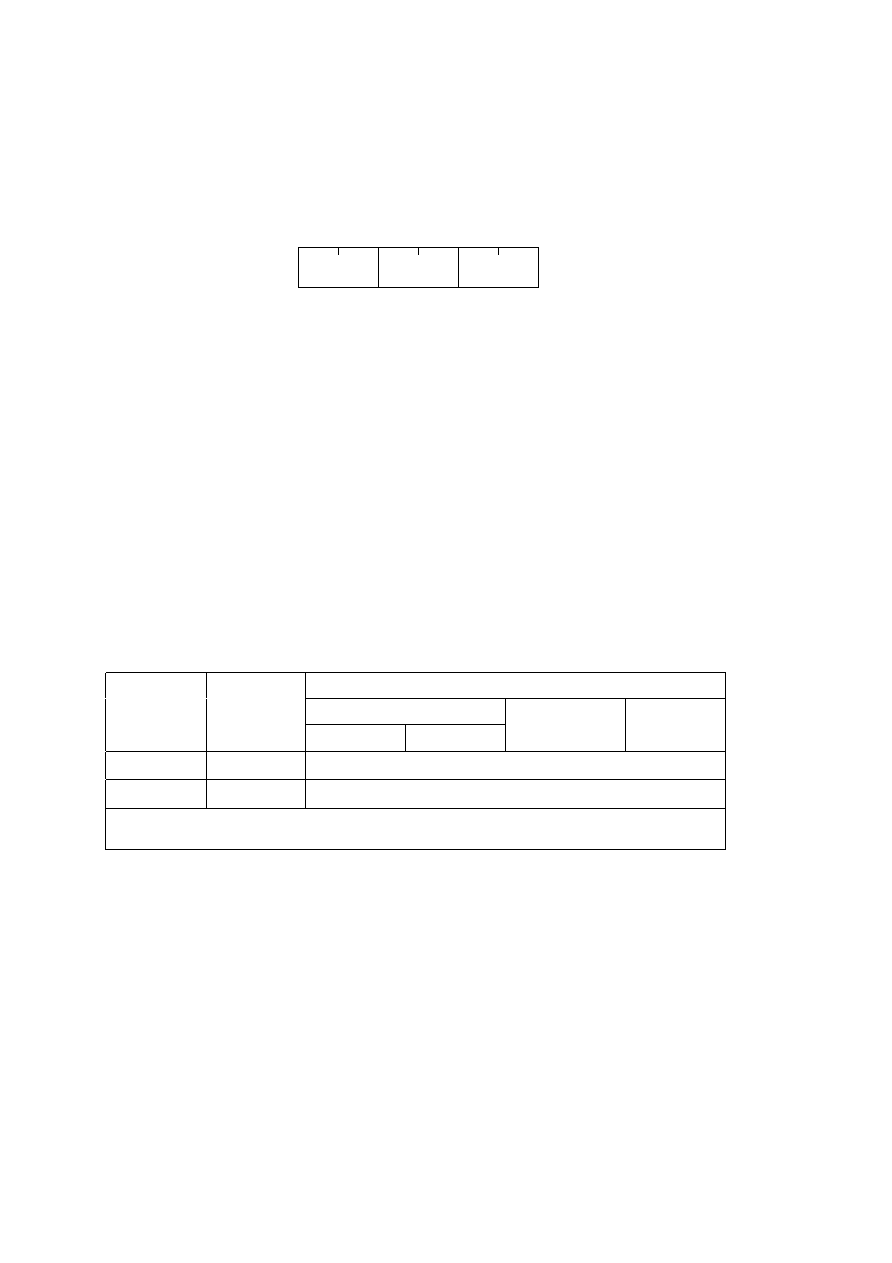ISO/IEC 10918-1 : 1993(E)
B.2.5
Define number of lines syntax
Figure B.12 specifies the marker segment for defining the number of lines. The DNL (Define Number of Lines) segment
provides a mechanism for defining or redefining the number of lines in the frame (the Y parameter in the frame header) at
the end of the first scan. The value specified shall be consistent with the number of MCU-rows encoded in the first scan.
This segment, if used, shall only occur at the end of the first scan, and only after coding of an integer number of MCU-
rows. This marker segment is mandatory if the number of lines (Y) specified in the frame header has the value zero.
DNL
Ld
NL
TISO0940-93/d030
Define number of lines segment
Figure B.12 ­ Define number of lines syntax
Figure B.12 [D30], = 2.8 cm = 98.%
The marker and parameters shown in Figure B.12 are defined below. The size and allowed values of each parameter are
given in Table B.10.
DNL:
Define number of lines marker ­ Marks the beginning of the define number of lines segment.
Ld:
Define number of lines segment length ­ Specifies the length of the define number of lines segment shown
in Figure B.12 (see B.1.1.4).
NL:
Number of lines ­ Specifies the number of lines in the frame (see definition of Y in B.2.2).
Table B.10 ­ Define number of lines segment parameter sizes and values
Values
Parameter
Size (bits)
Sequential DCT
Progressive DCT
Lossless
Baseline
Extended
Ld
16
4
-65535
a)
NL
16
1-65 535
a)
a)
The value specified shall be consistent with the number of lines coded at the point where the DNL segment
terminates the compressed data segment.
B.3
Hierarchical syntax
B.3.1
High level hierarchical mode syntax
Figure B.13 specifies the order of the high level constituent parts of the interchange format for hierarchical encoding
processes.
CCITT Rec. T.81 (1992 E)
45